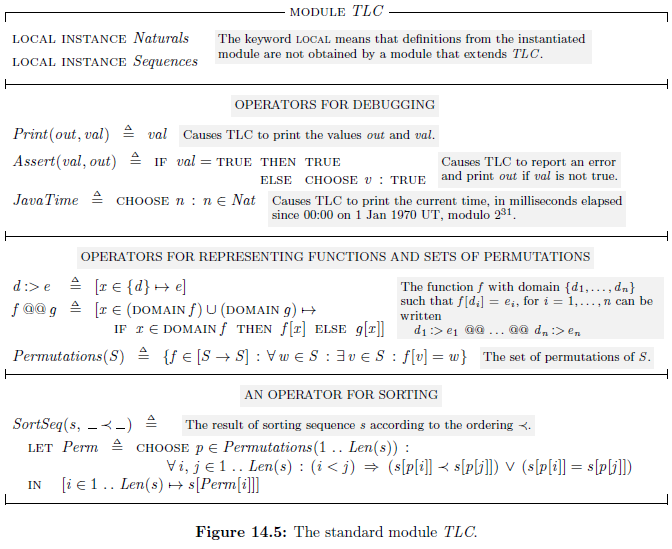《Specifying Systems》 阅读笔记。

## 1. A Little Simple Math

### 1.1. Propositional Logic

Propositional Logic (命题逻辑) is the mathematics of the two Boolean values TRUE and FALSE and the five operators whose names are:

• $\land$ conjunction (and)
• $\lor$ disjunction (or)
• $\lnot$ negation (not)
• $\Rightarrow$ implication (implies)
• $\equiv$ equivalence (is equivalent to)

Tautology (恒真式) is a formula or assertion that is ture in every possible interpretation. 比如 $F \Rightarrow G \equiv \lnot F \lor G$ 是一个 tautology。

### 1.2. Predicate Logic

Predicate Logic (谓词逻辑) extends propositional logic with the two quantifiers:

• $\forall$ universal quantification (for all)
• $\exists$ existential quantification (there exists)

## 2. Specifying a Simple Clock

• state: an assignment of values to varibles.
• behavior is an infinite sequence of states.
• step: a pair of successive states.
• action: an action is a formula, is true or false of a step.
• A step is satisfies the action $A$ is called an $A$ step
• An action is a formula, and formulas aren’t executed
• stuttering steps: steps that leave specified state(vars) unchanged.
• In TLA, we let $[Next]_{vars}$ stand for $Next \lor (vars' = vars)$
• theorem: a temporal formula satisfied by every behavior is called a theorem.

### 2.1. 小结

1. 先给出系统初始状态 $Init$
2. 再描述系统状态变化的行为 $Next$
3. 然后，得到 $Spec \triangleq Init \land \Box [Next]_{vars}$
4. 最后给出系统应满足的条件：$\mathrm{THEOREM} \enspace Spec \Rightarrow \mathrm{Temporal \enspace formula}$

## 3. An Asynchronous Interface

### 3.1. Invariant & Type

• A state function is an ordinary expression (one with no prime or $\Box$) that can contain variables and constants.
• A state predicate is a Boolean-valued state function. (one with no prime or $\Box$)
• An invariant $Inv$ of a specification $Spec$ is a state predicate such that $Spec \Rightarrow \Box Inv$ is a theorem.
• A variable $v$ has type $T$ in a specification $Spec$ iff $v \in T$ is an invariant of $Spec$.
• PS: TLA+ is an untyped language. Type correctness is just a name for a certain invariance property.

### 3.2. Record

• A record is a function whose domain is a finite set of strings.

• eg. $[val \mapsto d, ack \mapsto 1, rdy \mapsto 0]$ can be written

$[i \in \lbrace “val”, “ack”, “rdy”\rbrace \mapsto$ $$\mathrm{IF} \enspace i = “val” \enspace \mathrm{THEN} \enspace d \enspace \mathrm{ELSE \enspace IF} \enspace i = “ack” \enspace \mathrm{THEN} \enspace 1 \enspace \mathrm{ELSE} \enspace 0]$$

• The set of records is written: $[val : Data, rdy : \{0, 1\}, ack : \{0, 1\}]$

• The fields of a record are not ordered

• In general, for any record $r$, the expression $[r \enspace \mathrm{EXCEPT} \enspace !.c_1 = e_1, \cdots , !.c_n = e_n]$ is the record obtained from $r$ by replacing $r.c_i$ with $e_i$, for each $i$ in $1 .. n$.

• ! as standing for the new record $r'$
• an @ in the expression $e_i$ stands for $r.c_i$

### 3.3. Definitions

• operators: We define operators that take more than one argument in the obvious way, the general form being

$$Id(p_1, \dots, p_n) \triangleq exp$$

where the $p_i$ are distinct identifiers and $exp$ is an expression.

• symbol: mean an identifier or an operator.

• Every symbol declaration or definition has a scope within the symbol may bes used.

• The statement $\mathrm{EXTENDS}$ extends the scope of symbols

• A symbol cannot be declared or defined if it already has a meaning.

eg. $\exists v \in S : (exp1 \land \exists v \in T : exp2)$ is illegal.

• 单行注释：\*
• 多行注释：(*....*)

### 3.5. 小结

1. 可以通过定义 invariant 描述系统该满足的条件，以便于理解，比如 type-correctness invariant
2. 可以使用 record 将多个变量整合为单个变量

## 4. A FIFO

### 4.1. Instantiation Examined

Instantiation Is Substitution

Let’s now consider an arbitrary $\mathrm{INSTANCE}$ statement

$$IM \triangleq \mathrm{INTANCE} \enspace M \enspace \mathrm{WITH} \enspace p_1 \leftarrow e_1, \dots, p_n \leftarrow e_n$$

Let $\Sigma$ be a symbol defined in module $M$ and let $d$ be its “real” definition.

• The $\mathrm{INSTANCE}$ statement defines $IM!\Sigma$ to have as its real definition the expression obtained from $d$ by replacing all instances of $p_i$ by the expression $e_i$, for each $i$.
• The definition of $IM!\Sigma$ must contain only the parameters (declared constants and variables) of the current module, not the ones of module $M$.
• Hence, the $p_i$ must consist of all the parameters of module $M$.
• The $e_i$ must be expressions that are meaningful in the current module.

Parametrized Instantiation

$$InChan \triangleq \mathrm{INTSANCE} \enspace Channel \enspace \mathrm{WITH} \enspace Data \leftarrow Message, chan \leftarrow in$$ $$OutChan \triangleq \mathrm{INTSANCE} \enspace Channel \enspace \mathrm{WITH} \enspace Data \leftarrow Message, chan \leftarrow out$$

$$Chan(ch) \triangleq \mathrm{INTSANCE} \enspace Channel \enspace \mathrm{WITH} \enspace Data \leftarrow Message, chan \leftarrow ch$$

Implicit Substitutions

$$InChan \triangleq \mathrm{INTSANCE} \enspace Channel \enspace \mathrm{WITH} \enspace Data \leftarrow Data, chan \leftarrow in$$

TLA+ allows us to drop any substiotution of the form $\Sigma \leftarrow \Sigma$, for a symbol $\Sigma$. So, the statement above can be written as

$$InChan \triangleq \mathrm{INTSANCE} \enspace Channel \enspace \mathrm{WITH} \enspace chan \leftarrow in$$

Instantiation Without Renaming

$$\mathrm{INTSANCE} \enspace Channel \enspace \mathrm{WITH} \enspace Data \leftarrow D, chan \leftarrow x$$

This instantiates $Channel$ with no renaming, but with substitution.

### 4.2. Hiding variables

In TLA, we hide a variable with the existential quantifier of temporal logic.

• In the formula $x : F$, the symbol $x$ is declared to be a variable in $F$.
• Unlike $\exists r : F$, which asserts the existence of a single value $r$, the formula $x : F$ asserts the exitence of a value for $x$ in each state of a behavior.

$Inner(q) \triangleq \mathrm{INSTANCE} \enspace InnerFIFO$

$Spec \triangleq$ $q : Inner(q)!Spec$

The variable parameter $q$ of module $InnerFIFO$ is instantiated with the parameter $q$ of the definition of $Inner$.

### 4.3. ASSUME

• An $\mathrm{ASSUME}$ statement should be used only to make assumptions about constants
• 我们不需要假定某个常量是集合类型，because in TLA+, every value is a set.

### 4.4. 小结

1. 介绍了 Instantiation，并展示了如何利用实例化以使用其他 module
2. 通过利用量词 和参数实例化的特性可达到隐藏变量的目的
3. 利用 $\mathrm{ASSUME}$ 语句可以设定 $\mathrm{CONSTANT}$ 的取值范围

## 5. A Caching Memory

### 5.1. Parameters

• The way to leave something unspecified in a specification is to make ti a parameter

• TLA+ provides only $\mathrm{CONSTANCE}$ and $\mathrm{VARIABLE}$ parameters, not action parameters, we can declare a constant operator to descirbe the action.

• In TLA+, we declare $Send$ to be a constant operator that takes four arguments by writing:

$$\mathrm{INSTANCE} \enspace Send(\rule[-0pt]{0.3cm}{0.5pt}， \rule[-0pt]{0.3cm}{0.5pt}， \rule[-0pt]{0.3cm}{0.5pt}， \rule[-0pt]{0.3cm}{0.5pt})$$

• The expression $\mathrm{CHOOSE} \enspace x : F$ equals an arbitrarily chosen value $x$ that satisfies the formula $F$. If no such $x$ exists, the expression has a completely arbitrary value.

• The follow statement defines $NoVal$ to be some value this is not an element of $Val$. $$NoVal \triangleq \mathrm{CHOOSE} \enspace v : v \notin Val$$

### 5.2. Functions

• A function $f$ has a domain, written $\mathrm{DOMAIN} \enspace f$, and it assigns to each element $x$ of its domain the value $f[x]$

• For any sets $S$ and $T$, the set of all functions whose domain equals $S$ and whose range is any subset of $T$ is written $[S \rightarrow T]$

• A record is a function whose domain is a finite set of strings

• In general, $[f \enspace \mathrm{EXCEPT} \enspace ![c_1] = e_1, \dots, ![c_n] = e_n]$ is the function $\hat{f}$ that is the same as $f$ except with $\hat{f}[c_i]= e_i$ for each $i$. More precisely, this expression equals

$$[\dots[[f \enspace \mathrm{EXCEPT} \enspace ![c_1] = e_1]\enspace \mathrm{EXCEPT} \enspace ![c_2] = e_2]\dots \enspace \mathrm{EXCEPT} \enspace ![c_n] = e_n]$$

• Functions correspond to the arrays of programming languages. A function whose range is a set of functions corresponds to an array of arrays. eg:

$$[f \enspace \mathrm{EXCEPT} \enspace ![c][d] = e]$$

can be written as

$$[f \enspace \mathrm{EXCEPT} \enspace ![c] = [@ \enspace \mathrm{EXCEPT} \enspace ![d] = e]]$$

• TLA+ uniformly maintains the notation that $\sigma.c$ is an abbreviation for $\sigma[“c”]$. For example, this implies

$$[f \enspace \mathrm{EXCEPT} \enspace ![c].d = e] = [f \enspace \mathrm{EXCEPT} \enspace ![c][“d”] = e]$$

• In TLA+, as in ordinary mathematics, a function of multiple arguments is one whose domain is a set of tuples. For example, $f[5, 3, 1]$ is an abbreviation for $f[\langle 5, 3, 1 \rangle]$, the value of the function $f$ applied to the triple $\langle 5, 3, 1 \rangle$

• TLA+ provides the Cartesian product operator $\times$ of ordinary mathematics, where $A \times B \times C$ is the set of all 3-tuples $\langle a, b, c \rangle$ such that $a \in A, b \in B$, and $c \in C$.

• The $\mathrm{LET}$ clause consists of a sequence of definitions whose scope extends until the end of the $\mathrm{IN}$ clause.

• When writing specifications, we almost always want to specify the new value of a variable $f$ rather than the new values of $f[i]$ for all $i$ in some set. $$\text{(a)} \space f' = [i \in Nat \mapsto i + 1] \qquad \surd$$ $$\text{(b)} \space \forall i \in Nat : f'[i] = i + 1 \qquad \times$$

• Formula (a) uniquely determines $f'$, asserting that it’s a function with domain $Nat$
• Formula (b) is satisfied by lots of different values of $f'$.In fact, wo can’t even deduce that $f'$ is a function.

Recursive function

• Follow definition is illegal because the occurrence of fact to the right of the $\triangleq$ is undefined $$fact \triangleq [n \in Nat \mapsto \mathrm{IF} \enspace n = 0 \enspace \mathrm{THEN} \enspace 1 \enspace \mathrm{ELSE} \enspace n * fact[n-1]]$$

• In general, a definition of the form $f[x \in S] \triangleq e$ can be used to define recursively a function $f$ with domain $S$.

• In general, $f[x \in S] \triangleq e$ is an abbreviation for $$f \triangleq \mathrm{CHOOSE} \enspace f : f = [x \in S \mapsto e]$$

• TLA+ does not allow mutually recursive definition, but you can convert any mutually recursive definitions into a single recursive definition of a record-valued function whose fields are the desired functions.(P68-69, 6.3)

### 5.3. Invariance

• In general, when $P \land N \Rightarrow P'$ holds, we say that predicate $P$ is an invariant of action $N$

### 5.4. 小结

1. 当不知如何规范化描述某事物时，可先将其看作 parameter
2. 可以利用 $\mathrm{CHOOSE}$ 表示不属于某集合的值
3. invariant of action $\neq$ invariant of formula

## 6. Some More Math

### 6.1. Sets

built-in operators of TLA+

• The most common operations:

• intersection $\cap$
• union $\cup$
• subset $\subseteq$
• set difference $\setminus$
• $\mathrm{UNION} \enspace S$: The union of the elements of $S$. For example, $$\mathrm{UNION} \enspace \lbrace \lbrace 1, 2 \rbrace, \lbrace 2, 3 \rbrace, \lbrace 3, 4 \rbrace \rbrace = \lbrace 1, 2, 3, 4 \rbrace$$

• $\mathrm{SUBSET} \enspace S$: The set of all subsets of $S$. For example, $$\mathrm{SUBSET} \enspace \lbrace 1, 2 \rbrace = \lbrace \lbrace \rbrace, \lbrace 1 \rbrace, \lbrace 2 \rbrace, \lbrace 1, 2 \rbrace \rbrace$$

• $\{x \in S : p\}$: The subset of $S$ consisting of all elements $x$ satisfying property $p$

• $\{e : x \in S\}$: The set of elements of the form $e$, for all $x$ in the set $S$

Let $\mathcal{R}$ be the set of all sets $S$ such that $S \notin S$. The definiton of $\mathcal{R}$ implies that $\mathcal{R} \in \mathcal{R}$ is true iff $\mathcal{R} \notin \mathcal{R}$ is true.

A collection $\mathcal{C}$ is too big to be a set if it is as big as the collection of all sets — meaning that we can assign to every set a different element of $\mathcal{C}$. That is, $\mathcal{C}$ is too big to be a set if we can define an operator $SMap$ such that

• $SMap(S)$ is in $\mathcal{C}$, for any set $S$
• If $S$ and $T$ are two different sets, then $SMap(S) \neq SMap(T)$

### 6.2. Silly Expressions

• TLA+ is based on the usual formalization of mathematics by mathematicians, which doesn’t hava types.
• A correct formula can contain silly expressions.

### 6.3. Functions versus Operators

$$Tail(s) \triangleq [i \in 1 .. (Len(s) - 1) \mapsto s[i+1]]$$ $$fact[n \in Nat] \triangleq \mathrm{IF} \enspace n = 0 \enspace \mathrm{THEN} \enspace 1 \enspace \mathrm{ELSE} \enspace n*fact[n-1]$$

• A function like $fact$ by itself is a complete expression that denote a value, but an operator like $Tail$ is not.
• Unlike an operator, a function must have a domain, which is a set.
• Unlike a function, an operator cannot be defined recursively in TLA+.

However, we can usually transform an illegal recursive operator definition into a nonrecursive one using a recursive function definition.

• Operators also differ from functions in that an operator can take an operator as an argument.
• The definition of $Tail$ defines $Tail(s)$ for all values of $s$. whatever $Tail(s)$ means, it equals $Tail(s)$. But the definition of $fact$ defines $fact[n]$ only for $n \in Nat$, it tells us nothing about the value of $fact[1/2]$.
• TLA+ doesn’t permit us to define infix functions

## 7. Writing a Specification: Some Advice

• Why Specify?
• help the design process
• consice way of communicating a design
• find errors in the design and to help in testing the system
• What to Specify?
• TLA+ is particularly effective at revealing concurrency errors - ones that arise through the interraction of asynchronous components. So when writing a TLA+ specification, you will probably concentrate your efforts on the parts of the system that are most likely to have such errors.
• The level of abstraction — tradeoff of precise and cost
• The Grain of Atomicity
• The Data Structures
• Writing the Specification
1. Pick the variables and define the type invariant and initial predicate
2. Write the next-state action, which forms the bulk of the specification
3. Write the temporal part of the specification
4. Assert theorems about the specification
• Some Further Hints
• Don’t be too clever, make a specification easy to read
• A type invariant is not an assumption, is a property of a specification
• Don’t be too abstract
• Don’t assume values that look different are unequal
• The rules of TLA+ do not imply that $1 \neq “a”$
• Move quantification to the outside
• Prime only what you mean to prime
• PS: you can prime only an expression, not an operator

## 8. Liveness and Fairness

• Safety properties: what a system must not do
• Liveness properties: something does happen
• We express liveness properties as temporal formulas
• Liveness properties are likely to be the least important part of your specification

### 8.1. Temporal Formulas

Definition

• A temporal formula is true or false of a $behavior$.
• Formally, a temporal formula $F$ assigns a Boolean value, which we write $\sigma \models F$, to a behavior $\sigma$.
• We say that $F$ is true of $\sigma$, or that $\sigma$ satisfies $F$, iff $\sigma \models F$ equals $\mathrm{TRUE}$.

PS: reviews

Term A formula is true or false of this term
state state predicate $P$
setp action $A$
behavior temporal formula $F$

Unquantified temporal formuals

All the unquantified temporal formulas that we’ve seen have been Boolean combinations of three simple kinds of formulas:

1. A $state \ predicate$ $P$, viewed as a temporal formula, is true of a behavior iff it is true in the first state of the behavior.
2. A formula $\Box P$, where $P$ is a $state \ predicate$, is true of a behavior iff $P$ is true in every state of the behavior
3. A formula $\Box [N]_v$, where $N$ is an $action$ and $v$ is a state function, is true of a behavior iff every successive pair of steps in the behavior is a $[N]_v$ step.

Since a state predicate is an action that contains no primed variables, than $$\{P, \Box P, \Box [N]_v\} \equiv \{A, \Box A\}$$ where $A$ is an action.

• We interpret an arbitrary action $A$ as a temporal formula by defining $\sigma \models A$ to be true iff the first two states of $\sigma$ are an $A$ step
• use the notation $\sigma_i$ that is the $(i+1)^{st}$ state of the behavior $\sigma$, for any natural number $i$, so $\sigma$ is the behavior $\sigma_0 \rightarrow \sigma_1 \rightarrow \sigma_2 \rightarrow \cdots$
• That is, $\sigma \models A$ is true iff $\sigma_0 \rightarrow \sigma_1$ is an $A$ step
• We defined $\sigma \models \Box A$ to be true iff $\sigma_n \rightarrow \sigma_{n+1}$ is an $A$ step for all $n$. In other words: $$\sigma \models \Box A \quad \equiv \quad \forall n \in Nat : \sigma^{+n} \models A$$ where $\sigma^{+n} \triangleq \sigma_n \rightarrow \sigma_{n+1} \rightarrow \sigma_{n+2} \rightarrow \cdots$

The obvious generalization is

$$\sigma \models \Box F \quad \triangleq \quad \forall n \in Nat : \sigma^{+n} \models F$$

for any temporal formula $F$. In other words, $\sigma$ satisfies $\Box F$ iff every $\sigma^{+n}$ of $\sigma$ satisfies $F$.

• This defines the meaning of the temporal operator $\Box$. We can therefore read $\Box$ as always or henceforth or from then on.
• We say that a formula $F$ is invariant under stuffering iff adding or deleting a stuffering step to a behavior $\sigma$ does not affect whether $\sigma$ satisfies $F$
• TLA allows you to write only temporal formulas that are invariant under stuttering
• A state predicate (viewed as a temporal formula) is invariant under stuttering
• In general, the formula $\Box [A]_v$ is invariant under stuttering, for ant action $A$ and state function $v$. However, $\Box A$ is not invariant under stuttering for an arbitrary action $A$
• Invariant under stuttering is preserved by $\Box$ and by Boolean operators — that is, if $F$ and $G$ are invariant under stuttering, then so are $\Box F$, $\lnot F$, $F \land G$, $\forall x \in S : F$, etc.

Five especially important classes of formulas

• $\Diamond F \quad$ is defined to equal $\lnot \Box \lnot F$. It asserts that $F$ is not always false, which means that $F$ is true at some time

$$\sigma \models \Diamond F \quad \equiv \quad \exists n \in Nat : \sigma^{+n} \models F$$

We usually read $\Diamond$ as eventually, taking eventually to include now.

• $F \rightsquigarrow G \quad$ is defined to equal $\Box(F \Rightarrow \Diamond G)$. It asserts that whenever $F$ is true, $G$ is eventually true — that is, $G$ is true then or at some later time.

$\sigma \models (F \rightsquigarrow G) \quad \equiv \quad$ $$\forall n \in Nat : (\sigma^{+n} \models F) \Rightarrow (\exists m \in Nat : (\sigma^{+(n+m)} \models G))$$

We read $\rightsquigarrow$ as leads to.

• $\Diamond \langle A \rangle_v \quad$ is defined to equal $\lnot \Box [\lnot A]_v$, where $A$ is an action and $v$ is a state function. It asserts that not every step is a $(\lnot A) \lor (v' = v)$ step, so some step is a $A \land (v' \ne v)$ — that is, an $A$ step that changes $v$. We defines the action $\langle A \rangle_v$ by

$$\langle A \rangle_v \triangleq A \land (v' \ne v)$$

so $\Diamond \langle A \rangle_v$ asserts that eventually $\langle A \rangle_v$ step occurs

• $\Box \Diamond F \quad$ asserts that at all times, $F$ is true then or at some later time.

• It asserts that $F$ is infinitely often true (无限但不连续)
• In particular, $\Box \Diamond \langle A \rangle_v$ asserts that infinitely many $\langle A \rangle_v$ steps occur
• $(\sigma \models \Box \Diamond F) \equiv (\exists_\infty i \in Nat : \sigma^{+i} \models F)$, where $\exists_\infty i \in Nat : P(i)$ means $P(i)$ is true for infinitely many natural numbers $i$
• $\Diamond \Box F \quad$ asserts that eventually (at some time), $F$ becomes true and remains true thereafter.

• It asserts $F$ is eventually always true (连续)
• In particular, $\Diamond \Box [N]_v$ asserts that, eventually, every step is a $[N]_v$ step

### 8.2. Temporal Tautologies

• A temporal theorem is a temporal formula that is satisfied by all behaviors.
• A formula like $\Box F \Rightarrow F$ that is true when any formulas are substituted for its identifiers is called a tautology, tautologies containing temporal operators are sometimes called temporal tautologies.

some temporal tautologies

A genneral law: from any temporal tautology, we obtain a dual tautology by making the replacements

$$\Box \leftarrow \Diamond \quad \Diamond \leftarrow \Box \quad \land \leftarrow \lor \quad \lor \leftarrow \land$$

and reversing the direction of all implications. (Any $\equiv$ or $\lnot$ is left unchanged.)

1. $\lnot \Box F \equiv \Diamond \lnot F$

• $F$ is not always true iff it is eventually false.
2. $\Box(F \land G) \equiv (\Box F) \land (\Box G) \qquad$

• $F$ and $G$ are both always true iff $F$ is always true and $G$ is always true
• $\Box$ distributes over $\land$
3. $\Diamond (F \lor G) \equiv (\Diamond F) \lor (\Diamond G)$

• $F$ or $G$ is eventually true iff $F$ is eventually true or $G$ is eventually true
• $\Diamond$ distributes over $\lor$
4. $(\Box F) \lor (\Box G) \Rightarrow \Box(F \lor G) \qquad \Diamond (F \land G) \Rightarrow (\Diamond F) \land (\Diamond G)$

• One can be derived from the derived from the another by substituting $\lnot F$ for $F$ and $\lnot G$ for $G$
5. $\Box \Diamond (F \lor G) \equiv (\Box \Diamond F) \lor (\Box \Diamond G) \qquad \Diamond \Box(F \land G) \equiv (\Diamond \Box F) \land (\Diamond \Box G)$

• $\Box \Diamond$ distributes over $\lor$ and $\Diamond \Box$ distributes over $\land$ (取决于右边的符号)

In any TLA tautology, replacing a temporal formula by an action yields a tautology — a formula that is true for all behaviors — even if that formula isn’t a legal TLA formula. For example, let’s substitue $\langle A \rangle_v$ and $\langle B \rangle_v$ for $F$ and $G$ in $5$ to get

$$\Box \Diamond (\langle A \rangle_v \lor \langle B \rangle_v) \quad \equiv \quad (\Box \Diamond \langle A \rangle_v) \lor (\Box \Diamond \langle B \rangle_v)$$

but $(\langle A \rangle_v \lor \langle B \rangle_v)$ isn’t a TLA formula (TLA allows you to write only temporal formulas that are invariant under stuttering). We can apply the following rules of logic to transform those nonTLA tautologies into TLA tautologies:

$$[A \land B]_v \quad \equiv \quad [A]_v \land [B]_v \qquad \qquad \langle A \lor B \rangle_v \quad \equiv \quad \langle A \rangle_v \lor \langle B \rangle_v$$

Substituting $\langle A \rangle_v \lor \langle B \rangle_v$ for $\langle A \lor B \rangle_v$, gives us the following TLA tautology:

$$\Box \Diamond \langle A \lor B \rangle_v \quad \equiv \quad (\Box \Diamond \langle A \rangle_v) \lor (\Box \Diamond \langle B \rangle_v)$$

### 8.3. Temporal Proof Rules

A proof rule is a rule for deducing true formulas from other true formulas.

Modus Ponens Rule

• for any formulas $F$ and $G$, if we have proved $F$ and $F \models G$, then we can deduce $G$
• the laws of propositional logic hold for temporal logic as well

Temporal Proof Rules

• Modus Ponens Rule
• Generalization Rule: From $F$ we can infer $\Box F$, for any temporal formula $F$
• This rule asserts that, if $F$ is true for all behaviors, then so is $\Box F$
• Implies Generalization Rule: From $F \models G$ we can infer $\Box F \models \Box G$, for any temporal formulas $F$ and $G$.
• The Generalization Rule can be derived from the Implies Generalization Rule.

Difference between proof rule and tautology

• In propositional logic, every proof rule has a corressponding tautology.
• In temporal logic, a proof rule need not imply a tautology

### 8.4. Weak Fairness

When writing liveness properties, the syntax of TLA often forces us to write $\langle A \rangle_v$ instead of $A$, for some action $A$

Because if $A$ does allow stuttering steps, we want to require not that an $A$ step eventually occurs, but that a nonstuttering $A$ step occurs — that is, an $\langle A \rangle_v$ step.

• TLA+ defines $\quad \mathrm{ENABLED} \ A \quad$ to be the predicate that is true iff action $A$ is enabled.

• General liveness condition for an action $A$:

$$\Box(\mathrm{ENABLED}\langle A \rangle_v \Rightarrow \Diamond \langle A \rangle_v)$$

• Weaker formula $WF_v(A)$, defined to equal(3 种等价描述) — weak fairness on $A$

• If $A$ is enabled forever, then an $A$ step eventually occurs

$\Box(\Box \mathrm{ENABLED}\langle A \rangle_v \Rightarrow \Diamond \langle A \rangle_v)$

• $A$ is infinitely often disabled, or infinitely many $A$ steps occur

$\Box \Diamond (\lnot \mathrm{ENABLED}\langle A \rangle_v) \lor \Box \Diamond \langle A \rangle_v$

• If $A$ is eventually enabled forever, then infinitely many $A$ steps occur

$\Diamond \Box (\mathrm{ENABLED} \langle A \rangle_v) \Rightarrow \Box \Diamond \langle A \rangle_v$

## 9. The Standard Modules

### 9.1. Sequences Module

The Sequences module defines the following operators on sequences:

• $Seq(S)$

• The set of all sequence of elements of the set $S$
• For example, $\langle 3, 7 \rangle$ is an element of $Seq(Nat)$
• $Head(S)$

• The first element of sequence $s$
• For example, $Head(\langle 3, 7 \rangle)$ equals $3$
• $Tail(s)$

• The tail of sequence $s$, which consists of $s$ with its head removed
• For example, $Tail(\langle 3, 7 \rangle)$ equals $\langle 7 \rangle$
• $Append(s, e)$

• The sequence obtained by appending element $e$ to the tail of sequence $s$
• For example, $Append(\langle 3, 7 \rangle, 3)$ equals $\langle 3, 7, 3 \rangle$
• $s \circ t$

• The sequence obtained by concatenating the sequences $s$ and $t$
• For example, $\langle 3, 7 \rangle \circ \langle 3 \rangle$ euqals $\langle 3, 7, 3 \rangle$
• $Len(s)$

• The length og sequence $s$.
• $SubSeq(s, m, n)$

• The subsequence $\langle s[m], s[m+1], \dots, s[n] \rangle$ consisting of the $m^{th}$ through $n^{th}$ elements of $s$
• It is underfined if $m < 1$ or $n > Len(s)$, except that it equals the empty sequence if $m > n$
• $SelectSeq(s, Test)$

• The subsequence of $s$ consisting of the elements $s[i]$ such that $Test(s[i])$ equals $\mathrm{TRUE}$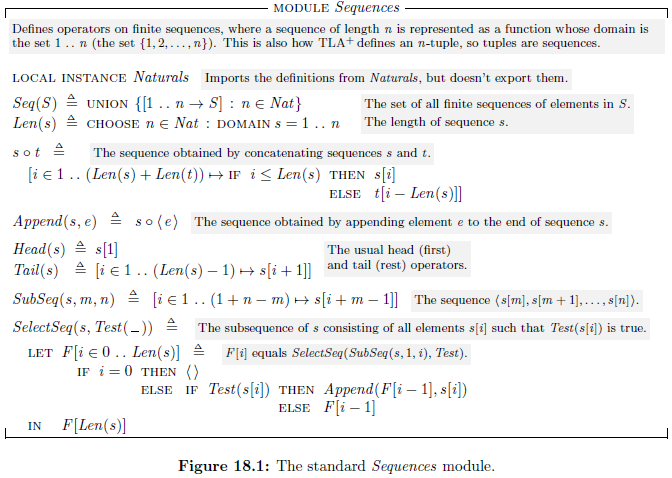### 9.2. FiniteSets Module

The FiniteSets module defines the following operators on set:

• $Cardinality(S)$

• The number of elements in set $S$, if $S$ is a finite set
• $IsFiniteSet(S)$

• True iff $S$ is a finite set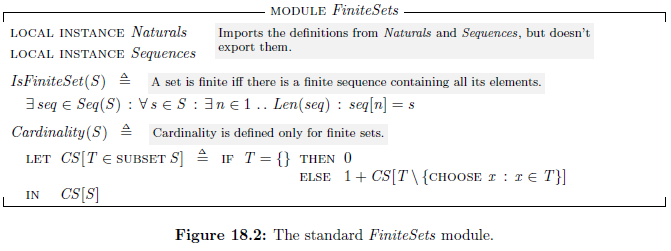### 9.3. Bags Module

A bag, also called a multiset, is a set that can contain multiple copies of the same element. A bag can have infinitely many elements, but only the finitely many copies of any single element.

The Bags module defines a bag to be a function whose range is a subset of the positive integers. The module defines the following operators:

• $IsABag(B)$

• True iff $B$ is a bag
• $BagToSet(B)$

• The set of elements of which bag $B$ contains at least one copy
• $SetToBag(S)$

• The bag that contains one copy of every element i nthe set $S$
• $BagIn(e, B)$

• True iff bag $B$ contains at least one copy of $e$.
• $BagIn$ is the $\in$ operator for bags
• $EmptyBag$

• The bag containing no elements
• $CopiesIn(e, B)$

• The number of copies of $e$ in bag $B • it is equal to$0$iff$BagIn(e, B)$is false •$B1 \oplus B2$• The union of bags$B1$and$B2$• The operator$\oplus$satifies $$CopiesIn(e, B1 \oplus B2) = CopiesIn(e, B1) + CopiesIn(e, B2)$$ for any$e$and any bags$B1$and$B2$•$B1 \ominus B2$• The bag$B1$with the elements of$B2$removed — that is, with one copy of an element removed from$B1$for each copy of the same element in$B2$• If$B2$has at least as many copies of$e$as$B1$, then$B1 \ominus B2$has no copies of$e$•$BagUnion(S)$• The bag union of all elements of the set$S$of bags. •$BagUnion$is the analog of$\mathrm{UNION}$for bags. • For example,$BagUnion(\{B1, B2, B3\})$equals$B1 \oplus B2 \oplus B3$•$B1 \sqsubseteq B2$• True iff, for all$e$, bag$B2$has at least as many copies of$e$as bag$B1$does •$\sqsubseteq$is the analog for bags of$\subseteq$•$SubBag(B)$• The set of all subbags of bag$B$•$SubBag$is the analog of$\mathrm{SUBSET}$for bags •$BagOfAll(F, B)$• It is thg bag that contains, for each element$e$of bag$B$, one copy of$F(e)$for every copy of$e$in$B$• This defines a bag iff, for any value$v$, the set of$e$in$B$such that$F(e) = v$is finite • The bag analog of the construct$\{F(x) : x \in B\}$•$BagCardinality(B)$• If$B$is a finite bag (one such that$BagToSet(B)$is a finite set), then this is its cardinality — the total number of copies of elements in$B$. • Its value is unspecified if$B\$ is not a finite bag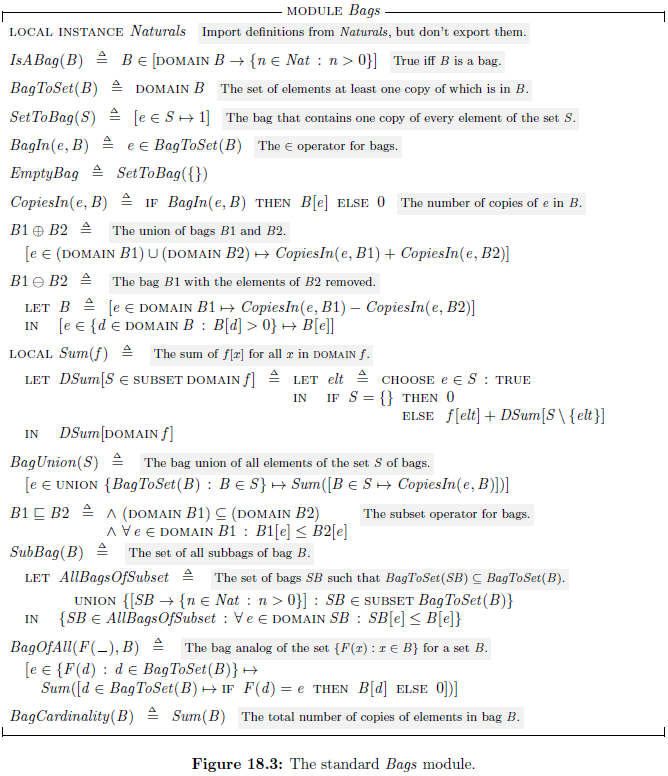### 9.4. The Numbers Modules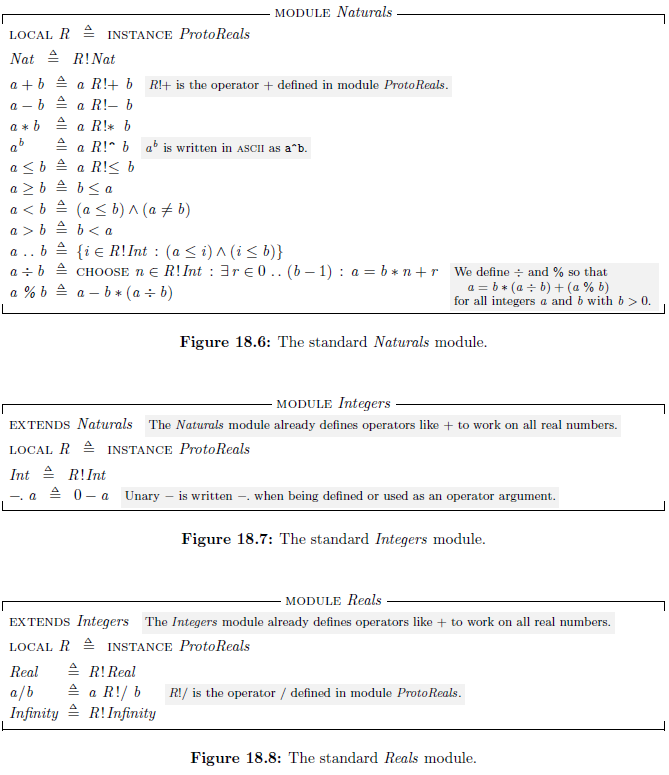### 9.5. RealTime Module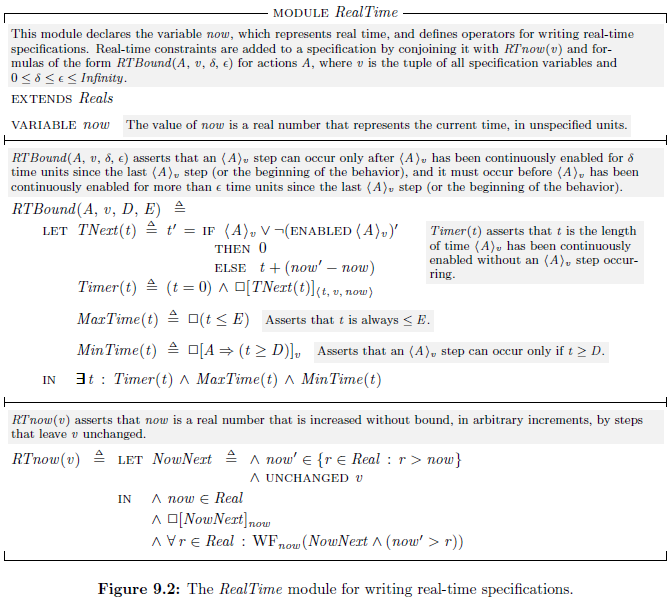### 9.6. TLC Module